• 双向循环神经网络可以双向传播，而循环神经网络只能前向传播。这意味着在处理时间序列数据时，可以前项数据会影响后项，后项也可以影响前项。
• 在训练过程中采用两个双向LSIM（ BILSIM）网络堆叠组成的模型，堆叠式的双向LSTM（ BILSIM）模型是一种改进的循环神经网络模型，相较于卷积神经网络，循环神经网络更加适合用来处理像心电图这样的序列数据。...深度学习算法神经网络
• 深度循环神经网络和双向循环神经网络 1、深度循环神经网络 本章到目前为止介绍的循环神经网络只有一个单向的隐藏层，在深度学习应用里，我们通常会用到含有多个隐藏层的循环神经网络，也称作深度循环神经网络。图...

深度循环神经网络和双向循环神经网络

动手学DL学习笔记

1、深度循环神经网络

本章到目前为止介绍的循环神经网络只有一个单向的隐藏层，在深度学习应用里，我们通常会用到含有多个隐藏层的循环神经网络，也称作深度循环神经网络。图6.11演示了一个有 个隐藏层的深度循环神经网络，每个隐藏状态不不断传递至当前层的下一时间步和当前时间步的下一层。

如下图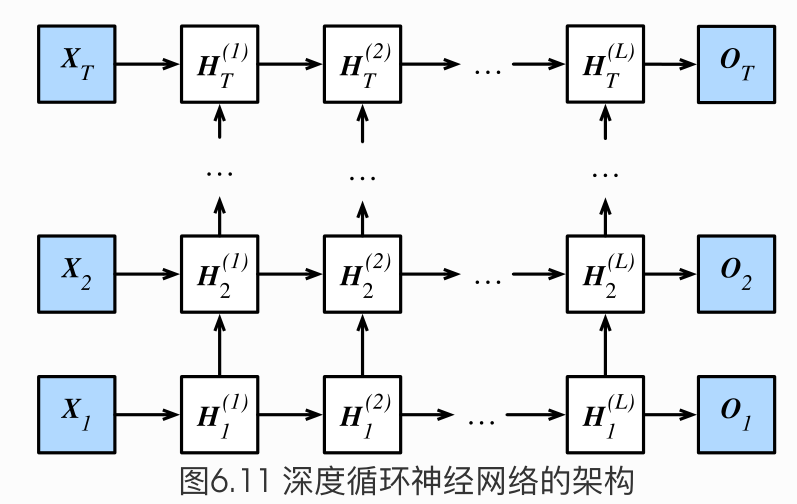H t ( 1 ) = ϕ ( X t W x h ( 1 ) + H t − 1 ( 1 ) W h h ( 1 ) + b h ( 1 ) ) H t ( ℓ ) = ϕ ( H t ( ℓ − 1 ) W x h ( ℓ ) + H t − 1 ( ℓ ) W h h ( ℓ ) + b h ( ℓ ) ) O t = H t ( L ) W h q + b q \boldsymbol{H}_t^{(1)} = \phi(\boldsymbol{X}_t \boldsymbol{W}_{xh}^{(1)} + \boldsymbol{H}_{t-1}^{(1)} \boldsymbol{W}_{hh}^{(1)} + \boldsymbol{b}_h^{(1)})\\\boldsymbol{H}_t^{(\ell)} = \phi(\boldsymbol{H}_t^{(\ell-1)} \boldsymbol{W}_{xh}^{(\ell)} + \boldsymbol{H}_{t-1}^{(\ell)} \boldsymbol{W}_{hh}^{(\ell)} + \boldsymbol{b}_h^{(\ell)})\\\boldsymbol{O}_t = \boldsymbol{H}_t^{(L)} \boldsymbol{W}_{hq} + \boldsymbol{b}_q
我们之前学的到的循环神经网络都单隐藏层的，如下图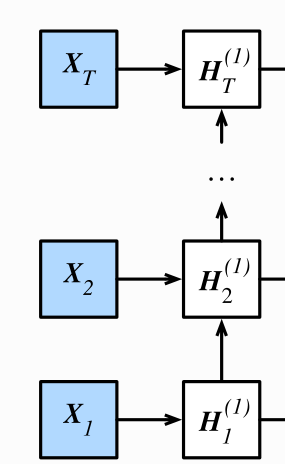• 具体来说就是，将X1输入到第一个循环神经元H1，这个rnn神经元可以是GRU也可以是LSTM，这里的H1是第一个时间步输出的隐藏状态，其将作为第二个时间步的输入隐藏状态，隐藏状态就这样一直往上传，直到HT。每个Hi（i=1,2,3…T）之后都会接一个输出层output（Oi i=1,2,3…T)，这就是之前的单隐层RNN。

• 对于深度循环神经网络，只是将第一层的每个隐藏状态Hi（i=1,2,3…T），做为第二层的输入，最后。H的上标表示层数，下标表示时间步数。在第L层之后做输出。

部分代码 加上num_layers即可食用 (两层的)

num_hiddens=256
num_epochs, num_steps, batch_size, lr, clipping_theta = 160, 35, 32, 1e2, 1e-2
pred_period, pred_len, prefixes = 40, 50, ['分开', '不分开']

#lr = 1e-2  注意调整学习率
#只需要在nn.LSTM原来的基础上加上参数num_layers=2,即可变成两层的循环神经网络(输入层+两个隐层+输出层)
gru_layer = nn.LSTM(input_size=vocab_size, hidden_size=num_hiddens,num_layers=2)
model = d2l.RNNModel(gru_layer, vocab_size).to(device)
d2l.train_and_predict_rnn_pytorch(model, num_hiddens, vocab_size, device,
corpus_indices, idx_to_char, char_to_idx,
num_epochs, num_steps, lr, clipping_theta,
batch_size, pred_period, pred_len, prefixes)

部分代码 (六层的)

gru_layer = nn.LSTM(input_size=vocab_size, hidden_size=num_hiddens,num_layers=6)
model = d2l.RNNModel(gru_layer, vocab_size).to(device)
d2l.train_and_predict_rnn_pytorch(model, num_hiddens, vocab_size, device,
corpus_indices, idx_to_char, char_to_idx,
num_epochs, num_steps, lr, clipping_theta,
batch_size, pred_period, pred_len, prefixes)

实践证明，上边num_layers=2的结果要比num_layers=6的结果要好很多的，所以说深度循环网络不是越深越好。

越深表示模型越复杂，对数据集的要求更高，内容更加抽象。所以参数的设置还要看实践和经验。

小结

• 在深度循环神经网络中，隐藏状态的信息不断传递至当前层的下一时间步和当前时间步的下一层。

2、双向循环神经网络

之前介绍的循环神经网络模型都是假设当前时间步是由前面的较早时间步的序列决定的，因此它们都将信息通过隐藏状态从前往后传递。有时候，当前时间步也可能由后⾯面时间步决定。例如，当我们写下一个句子时，可能会根据句子后面的词来修改句子前面的用词。双向循环神经网络通过增加从后往前传递信息的隐藏层来更灵活地处理这类信息。

图6.12演示了一个含单隐藏层的双向循环神经网络的架构。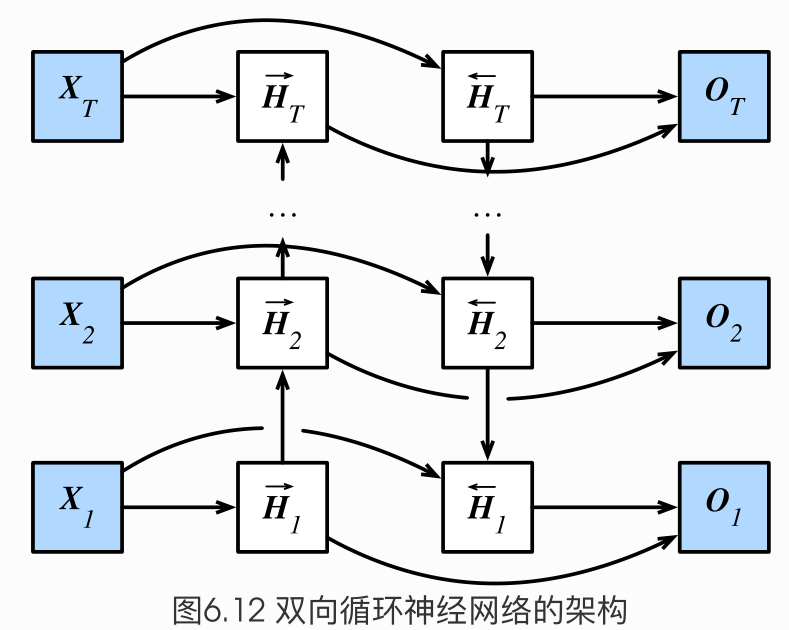H → t = ϕ ( X t W x h ( f ) + H → t − 1 W h h ( f ) + b h ( f ) ) H ← t = ϕ ( X t W x h ( b ) + H ← t + 1 W h h ( b ) + b h ( b ) ) \begin{aligned} \overrightarrow{\boldsymbol{H}}_t &= \phi(\boldsymbol{X}_t \boldsymbol{W}_{xh}^{(f)} + \overrightarrow{\boldsymbol{H}}_{t-1} \boldsymbol{W}_{hh}^{(f)} + \boldsymbol{b}_h^{(f)})\\\overleftarrow{\boldsymbol{H}}_t &= \phi(\boldsymbol{X}_t \boldsymbol{W}_{xh}^{(b)} + \overleftarrow{\boldsymbol{H}}_{t+1} \boldsymbol{W}_{hh}^{(b)} + \boldsymbol{b}_h^{(b)}) \end{aligned}

H t = ( H → t , H ← t ) \boldsymbol{H}_t=(\overrightarrow{\boldsymbol{H}}_{t}, \overleftarrow{\boldsymbol{H}}_t)

O t = H t W h q + b q \boldsymbol{O}_t = \boldsymbol{H}_t \boldsymbol{W}_{hq} + \boldsymbol{b}_q

• 我们可以分别计算正向隐藏状态（→）和反向隐藏状态（←）

• 两个方向的隐藏层的连接方式是 concat 拼接起来，蕴含了两个方向的信息 ，根据上图可以想成一个信息往上传，一个信息往下传。

• 不同方向上的隐藏单元个数也可以不同。

• 双向循环神经网络的好处：比如 X 1 到 X T 是一句话，本来我们只考虑了一个方向，即前面的字对后面的字影响。如果是双向的，那么每个字都会受到前面的字和后面的字的影响

很简单 加上 bidirectional=True 即可食用：

num_hiddens=128
num_epochs, num_steps, batch_size, lr, clipping_theta = 160, 35, 32, 1e-2, 1e-2
pred_period, pred_len, prefixes = 40, 50, ['分开', '不分开']

lr = 1e-2 # 注意调整学习率
#设置bidirectional=True 即可构建双向循环神经网络
gru_layer = nn.GRU(input_size=vocab_size, hidden_size=num_hiddens,bidirectional=True)
model = d2l.RNNModel(gru_layer, vocab_size).to(device)
d2l.train_and_predict_rnn_pytorch(model, num_hiddens, vocab_size, device,
corpus_indices, idx_to_char, char_to_idx,
num_epochs, num_steps, lr, clipping_theta,
batch_size, pred_period, pred_len, prefixes)

实践证明，双向带来了更多得参数，效果未必会好。

双向RNN是很常见的，但是否适用还需要实践来看效果。

小结

双向循环神经网络在每个时间步的隐藏状态同时取决于该时间步之前和之后的子序列（包括当前
时间步的输入）。

展开全文神经网络
• 这就需要双向循环神经网络（Bidirectional rnn）来解决这类问题。 二：结构 双向循环神经网络的主体结构是两个单向循环神经网络的结合。例如在某个时刻，输入会同时提供给这两个方向相反的循环神经网络，每个网络...

一：背景

在经典的循环神经网络中，状态的传输，是从前往后的，但是在有些任务中，某些时刻的输出不仅和 之前状态有关，也与之后的状态有关。这就需要双向循环神经网络（Bidirectional rnn）来解决这类问题。

二：结构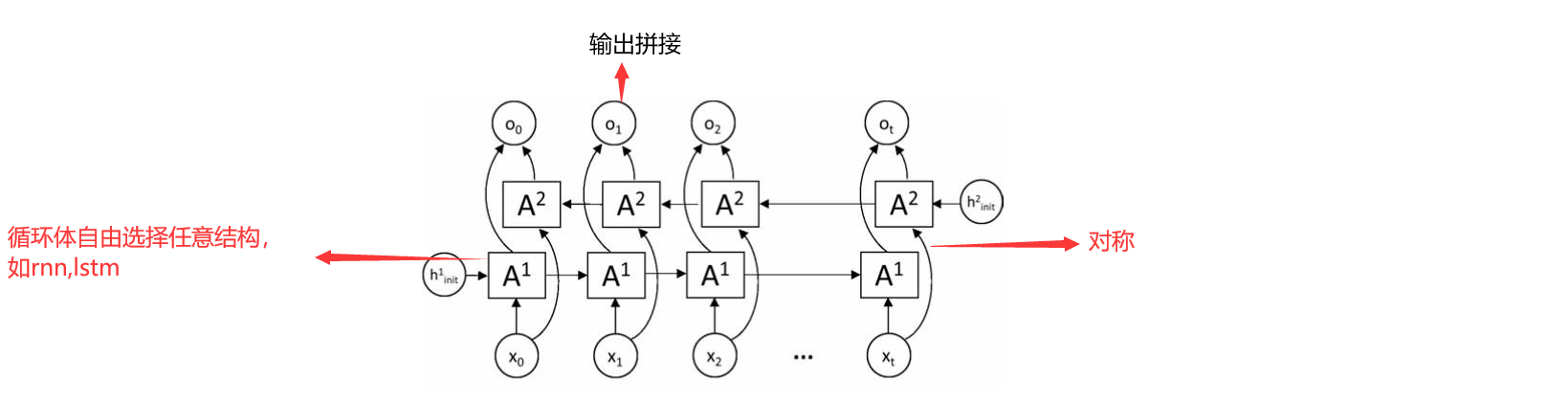双向循环神经网络的主体结构是两个单向循环神经网络的结合。例如在某个时刻，输入会同时提供给这两个方向相反的循环神经网络，每个网络生成各自的在该时刻的状态和输出，双向循环神经网络的输出会是由两个单向网络输出的简单拼接。除方向不一样外，其余结构完全相同。两个网络结构对称，任意网络的任意循环体都可以选择任意循环结构，如rnn,lstm等等。。双向循环神经网络的前向传播过程和单向的循环神经网络十分类似，这里不再赘述。

三：模型推导：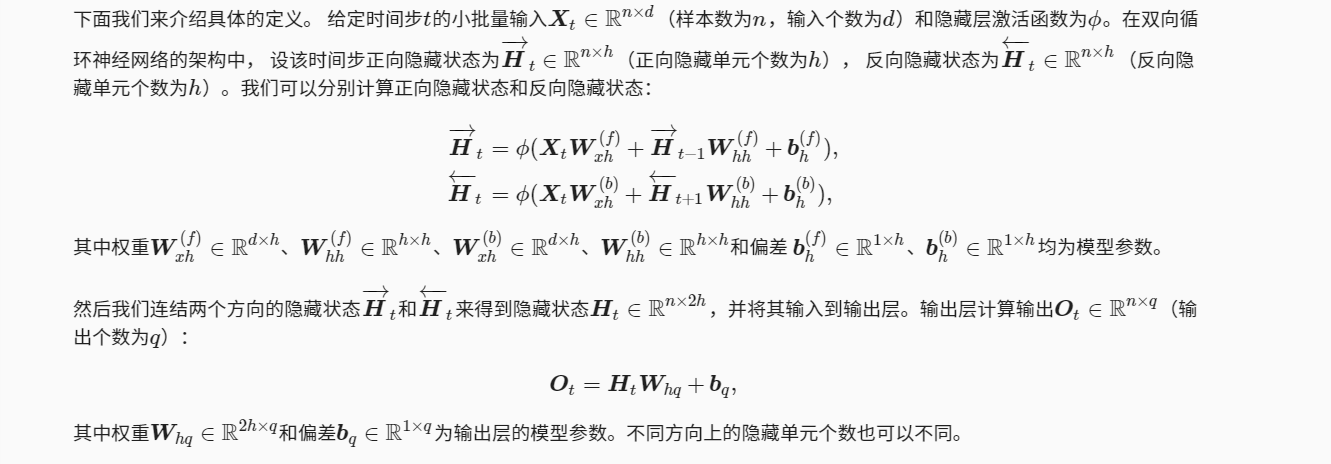展开全文神经网络
• 双向循环神经网络tensorflow代码，使用MNIST数据集，可直接运行
• MXNet双向循环神经网络----单个隐藏层的双向循环神经网络（程序） 《动手学深度学习》第六章 第10节的练习题，个人解答。 下图演示了一个含单隐藏层的双向循环神经网络的架构。 下面我们来介绍具体的定义。 给定...

MXNet双向循环神经网络----单个隐藏层的双向循环神经网络（程序）

《动手学深度学习》第六章 第10节的练习题，个人解答。

下图演示了一个含单隐藏层的双向循环神经网络的架构。下面我们来介绍具体的定义。
给定时间步 t t 的小批量输入 X t ∈ R n × d \boldsymbol{X}_t \in \mathbb{R}^{n \times d} （样本数为 n n ，输入个数为 d d ）和隐藏层激活函数为 ϕ \phi 。在双向循环神经网络的架构中，
设该时间步正向隐藏状态为 H → t ∈ R n × h \overrightarrow{\boldsymbol{H}}_t \in \mathbb{R}^{n \times h} （正向隐藏单元个数为 h h ），
反向隐藏状态为 H ← t ∈ R n × h \overleftarrow{\boldsymbol{H}}_t \in \mathbb{R}^{n \times h} （反向隐藏单元个数为 h h ）。我们可以分别计算正向隐藏状态和反向隐藏状态：

H → t = ϕ ( X t W x h ( f ) + H → t − 1 W h h ( f ) + b h ( f ) ) , H ← t = ϕ ( X t W x h ( b ) + H ← t + 1 W h h ( b ) + b h ( b ) ) , \begin{aligned} \overrightarrow{\boldsymbol{H}}_t &= \phi(\boldsymbol{X}_t \boldsymbol{W}_{xh}^{(f)} + \overrightarrow{\boldsymbol{H}}_{t-1} \boldsymbol{W}_{hh}^{(f)} + \boldsymbol{b}_h^{(f)}),\\ \overleftarrow{\boldsymbol{H}}_t &= \phi(\boldsymbol{X}_t \boldsymbol{W}_{xh}^{(b)} + \overleftarrow{\boldsymbol{H}}_{t+1} \boldsymbol{W}_{hh}^{(b)} + \boldsymbol{b}_h^{(b)}), \end{aligned}

其中权重 W x h ( f ) ∈ R d × h \boldsymbol{W}_{xh}^{(f)} \in \mathbb{R}^{d \times h} W h h ( f ) ∈ R h × h \boldsymbol{W}_{hh}^{(f)} \in \mathbb{R}^{h \times h} W x h ( b ) ∈ R d × h \boldsymbol{W}_{xh}^{(b)} \in \mathbb{R}^{d \times h} W h h ( b ) ∈ R h × h \boldsymbol{W}_{hh}^{(b)} \in \mathbb{R}^{h \times h} 和偏差 b h ( f ) ∈ R 1 × h \boldsymbol{b}_h^{(f)} \in \mathbb{R}^{1 \times h} b h ( b ) ∈ R 1 × h \boldsymbol{b}_h^{(b)} \in \mathbb{R}^{1 \times h} 均为模型参数。

然后我们连结两个方向的隐藏状态 H → t \overrightarrow{\boldsymbol{H}}_t H ← t \overleftarrow{\boldsymbol{H}}_t 来得到隐藏状态 H t ∈ R n × 2 h \boldsymbol{H}_t \in \mathbb{R}^{n \times 2h} ，并将其输入到输出层。输出层计算输出 O t ∈ R n × q \boldsymbol{O}_t \in \mathbb{R}^{n \times q} （输出个数为 q q ）：

O t = H t W h q + b q , \boldsymbol{O}_t = \boldsymbol{H}_t \boldsymbol{W}_{hq} + \boldsymbol{b}_q,

其中权重 W h q ∈ R 2 h × q \boldsymbol{W}_{hq} \in \mathbb{R}^{2h \times q} 和偏差 b q ∈ R 1 × q \boldsymbol{b}_q \in \mathbb{R}^{1 \times q} 为输出层的模型参数。不同方向上的隐藏单元个数也可以不同。

小结

• 双向循环神经网络在每个时间步的隐藏状态同时取决于该时间步之前和之后的子序列（包括当前时间步的输入）。

练习

• 参考上图设计含多个隐藏层的双向循环神经网络。
import d2lzh as d2l
from mxnet import nd
from mxnet.gluon import rnn

(corpus_indices, char_to_idx, idx_to_char,

初始化模型参数

创建公式中出现的参数，并初始化。

num_inputs, num_hiddens, num_outputs = vocab_size, 256, vocab_size
ctx = d2l.try_gpu()

def get_params():
def _one(shape):
return nd.random.normal(scale=0.01, shape=shape, ctx=ctx)

def _three():
return (_one((num_inputs, num_hiddens)),
_one((num_hiddens, num_hiddens)),
nd.zeros(num_hiddens, ctx=ctx))

W_xhf, W_hhf, b_hf = _three()  # 前向参数
W_xhb, W_hhb, b_hb = _three()  # 反向参数

# 输出层参数
W_hq = _one((2*num_hiddens, num_outputs))
b_q = nd.zeros(num_outputs, ctx=ctx)
# 附上梯度
params = [W_xhf, W_hhf, b_hf, W_xhb, W_hhb, b_hb,
W_hq, b_q]
for param in params:
return params

定义模型

根据两个方向的隐藏状态 H → t \overrightarrow{\boldsymbol{H}}_t H ← t \overleftarrow{\boldsymbol{H}}_t ，以及输出层计算输出 O t \boldsymbol{O}_t 的计算公式创建双向循环神经网络模型。

def init_bi_state(batch_size, num_hiddens, ctx):
return (nd.zeros(shape=(batch_size, num_hiddens), ctx=ctx),
nd.zeros(shape=(batch_size, num_hiddens), ctx=ctx),)

def birnn(inputs, state, params):
[W_xhf, W_hhf, b_hf,W_xhb, W_hhb, b_hb,
W_hq, b_q] = params
(Hf,Hb,) = state
outputs = []
for X in inputs:
Hf = nd.sigmoid(nd.dot(X, W_xhf) + nd.dot(Hf, W_hhf) + b_hf)
Hb = nd.sigmoid(nd.dot(X, W_xhb) + nd.dot(Hb, W_hhb) + b_hb)

H = nd.concat(Hf,Hb,dim=1)
Y = nd.dot(H, W_hq) + b_q
outputs.append(Y)
return outputs, (Hf, Hb,)

num_epochs, num_steps, batch_size, lr, clipping_theta = 160, 35, 32, 1e2, 1e-1  #lr设小后，没输出
pred_period, pred_len, prefixes = 40, 50, ['分开', '不分开']

中间测试

[W_xhf, W_hhf, b_hf,W_xhb, W_hhb, b_hb,W_hq, b_q] = get_params()
(Hf,Hb,) = init_bi_state(batch_size, num_hiddens, ctx)
Hf,Hb,W_xhf
H = nd.concat(Hf,Hb,dim=1)
H

[[0. 0. 0. ... 0. 0. 0.]
[0. 0. 0. ... 0. 0. 0.]
[0. 0. 0. ... 0. 0. 0.]
...
[0. 0. 0. ... 0. 0. 0.]
[0. 0. 0. ... 0. 0. 0.]
[0. 0. 0. ... 0. 0. 0.]]
<NDArray 32x512 @gpu(0)>

训练预测

d2l.train_and_predict_rnn(birnn, get_params, init_bi_state, num_hiddens,
vocab_size, ctx, corpus_indices, idx_to_char,
char_to_idx, False, num_epochs, num_steps, lr,
clipping_theta, batch_size, pred_period, pred_len,
prefixes)

epoch 40, perplexity 153.796754, time 1.32 sec
- 分开 我不 我不 我不 我不 我不 我不 我不 我不 我不 我不 我不 我不 我不 我不 我不 我不 我
- 不分开哼不能 我不能 我不 我不 我不 我不 我不 我不 我不 我不 我不 我不 我不 我不 我不 我不
epoch 80, perplexity 33.458324, time 1.34 sec
- 分开 我不能够不起 我不能够不起 我不能够不起 我不能够不起 我不能够不起 我不能够不起 我不能够不起
- 不分开始的我 我不能够不起 我不能够不起 我不能够不起 我不能够不起 我不能够不起 我不能够不起 我不能够
epoch 120, perplexity 10.509801, time 1.32 sec
- 分开 我 这里什么 不会B血 我想就这样牵着你的手 不会B血 我想就这样牵着你的手 不会B血 我想就这样
- 不分开不 我不能再想 我不能再想 我不能再想 我不能再想 我不能再想 我不能再想 我不能再想 我不能再想
epoch 160, perplexity 4.527863, time 1.29 sec
- 分开的可爱女人 坏坏的让我疯狂的可爱女人 坏坏的让我疯狂的可爱女人 坏坏的让我疯狂的可爱女人 坏坏的让我
- 不分开我爱你 一朵莫默默默离开这样打我妈妈这样牵着你的手不放开离开我 不能承受我已无处可躲可以演戏 快使用

展开全文神经网络 深度学习 算法
• 双向循环神经网络 一般，我们认为循环神经网络模型都是假设当前时间步是由前面的较早时间步的序列决定的，因此它们都将信息通过隐藏状态从前往后传递。 有时候，当前时间步也可能由后面时间步决定。 例如，当我们写...

双向循环神经网络

一般，我们认为循环神经网络模型都是假设当前时间步是由前面的较早时间步的序列决定的，因此它们都将信息通过隐藏状态从前往后传递。
有时候，当前时间步也可能由后面时间步决定
例如，当我们写下一个句子时，可能会根据句子后面的词来修改句子前面的用词。双向循环神经网络通过增加从后往前传递信息的隐藏层来更灵活地处理这类信息。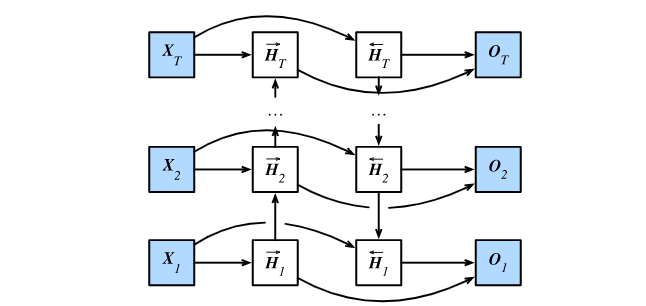• 给定时间步 t t 的小批量输入 X t ∈ R n × d \boldsymbol{X}_t \in \mathbb{R}^{n \times d} （样本数为 n n ，输入个数为 d d
• 隐藏层激活函数为 ϕ \phi

在双向循环神经网络的架构中， 设

• 该时间步正向隐藏状态为 H → t ∈ R n × h \overrightarrow{\boldsymbol{H}}_t \in \mathbb{R}^{n \times h} （正向隐藏单元个数为 h h
• 反向隐藏状态为 H ← t ∈ R n × h \overleftarrow{\boldsymbol{H}}_t \in \mathbb{R}^{n \times h} （反向隐藏单元个数为 h h ）。

我们可以分别计算正向隐藏状态和反向隐藏状态：

H → t = ϕ ( X t W x h ( f ) + H → t − 1 W h h ( f ) + b h ( f ) ) , H ← t = ϕ ( X t W x h ( b ) + H ← t + 1 W h h ( b ) + b h ( b ) ) \begin{aligned} \overrightarrow{\boldsymbol{H}}_t &= \phi(\boldsymbol{X}_t \boldsymbol{W}_{xh}^{(f)} + \overrightarrow{\boldsymbol{H}}_{t-1} \boldsymbol{W}_{hh}^{(f)} + \boldsymbol{b}_h^{(f)}),\\ \overleftarrow{\boldsymbol{H}}_t &= \phi(\boldsymbol{X}_t \boldsymbol{W}_{xh}^{(b)} + \overleftarrow{\boldsymbol{H}}_{t+1} \boldsymbol{W}_{hh}^{(b)} + \boldsymbol{b}_h^{(b)})\end{aligned}

其中权重 W x h ( f ) ∈ R d × h \boldsymbol{W}_{xh}^{(f)} \in \mathbb{R}^{d \times h} W h h ( f ) ∈ R h × h \boldsymbol{W}_{hh}^{(f)} \in \mathbb{R}^{h \times h} W x h ( b ) ∈ R d × h \boldsymbol{W}_{xh}^{(b)} \in \mathbb{R}^{d \times h} W h h ( b ) ∈ R h × h \boldsymbol{W}_{hh}^{(b)} \in \mathbb{R}^{h \times h} 和偏差 b h ( f ) ∈ R 1 × h \boldsymbol{b}_h^{(f)} \in \mathbb{R}^{1 \times h} b h ( b ) ∈ R 1 × h \boldsymbol{b}_h^{(b)} \in \mathbb{R}^{1 \times h} 均为模型参数。

然后通过连结两个方向的隐藏状态 H → t \overrightarrow{\boldsymbol{H}}_t H ← t \overleftarrow{\boldsymbol{H}}_t 来得到隐藏状态 H t ∈ R n × 2 h \boldsymbol{H}_t \in \mathbb{R}^{n \times 2h} ，并将其输入到输出层。

输出层计算输出 O t ∈ R n × q \boldsymbol{O}_t \in \mathbb{R}^{n \times q} （输出个数为 q q ）：

O t = H t W h q + b q , \boldsymbol{O}_t = \boldsymbol{H}_t \boldsymbol{W}_{hq} + \boldsymbol{b}_q,

其中权重 W h q ∈ R 2 h × q \boldsymbol{W}_{hq} \in \mathbb{R}^{2h \times q} 和偏差 b q ∈ R 1 × q \boldsymbol{b}_q \in \mathbb{R}^{1 \times q} 为输出层的模型参数。不同方向上的隐藏单元个数也可以不同。

展开全文深度学习 神经网络 算法
• 双向循环神经网络（Bidirectional RNN） 双向RNN模型（BRNN），可以在序列的某点处不但获取之前的信息，同时还可以获取这个序列点之后的信息，说的炫酷点就是get information from the future。 而标准的RNN ...循环神经网络 深度学习 人工智能
• 文章目录门控循环神经网络GRU长短期记忆LSTM深度循环神经网络双向循环神经网络 门控循环神经网络GRU 该网络的提出也同样是为了应对普通RNN的梯度问题 基本原理看这一篇就能懂：转载自知乎 简单来说，GRU就是通过一个...
• 使用Tensorflow 2.2 中的keras实现双向循环神经网络。训练速度非常慢。 import tensorflow as tf import numpy as np from tensorflow import keras import os os.environ['TF_CPP_MIN_LOG_LEVEL'] = '1' #读取本地...tensorflow
• 单向循环神经网络所谓的单向循环神经网络其实就是常见的循环神经网络，可以看到t时刻、t-1时刻、t+1时刻，不同时刻输入对应不同的输出，而且上一时刻的隐含层会影响当前时刻的输出。这种结构就是单向循环神经...
• 文章目录双向循环神经网络一、隐马尔可夫模型中的动态规划二、双向模型2.1 定义2.2 模型的计算成本及其应用三、双向循环神经网络的错误应用 双向循环神经网络   在序列学习中，我们以往假设的目标是：到目前为止，...深度学习 rnn
• 双向循环神经网络(BiRNN)

千次阅读 2020-09-19 09:31:39
双向循环神经网络（BRNN）的基本思想是提出每一个训练序列向前和向后分别是两个循环神经网络（RNN），而且这两个都连接着一个输出层。这个结构提供给输出层输入序列中每一个点的完整的过去和未来的上下文信息。下图...
• 前言 循环神经网络得益于其...所谓的单向循环神经网络其实就是常见的循环神经网络，可以看到t时刻、t-1时刻、t+1时刻，不同时刻输入对应不同的输出，而且上一时刻的隐含层会影响当前时刻的输出。这种结构就是单向循...
• tensorflow之双向循环神经网络

千次阅读 2017-06-01 14:20:58
一、定义尽管从多层感知器（MLP）到循环神经网络（RNN）的扩展看起来微不足道，但是这对于序列的学习具有深远意义。循环神经网络（RNN）的使用是用来处理序列数据的。在传统的神经网络模型中，层与层之间是全连接的...神经网络
• 针对前馈神经网络难以处理时序数据的问题,提出将双向循环神经网络(BiRNN)应用在自动语音识别声学建模中。首先,应用梅尔频率倒谱系数进行特征提取;其次,采用双向循环神经网络作为声学模型;最后,测试不同参数对系统...
• 所以最终的网络结构图如下，第一层为词嵌入层，第二层为双向循环神经网络层，正向网络的输出和反向网络的输出分别作为输入输到一个隐含层，最后再输入到 CRF 层。 分词标签 我们可以设定状态值集合S为(B,...分词 bilstm crf lstm 神经网络
• 双向循环神经网络（BRNN）

万次阅读 2018-07-27 21:19:24
双向循环神经网络（BRNN） RNN和LSTM都只能依据之前时刻的时序信息来预测下一时刻的输出，但在有些问题中，当前时刻的输出不仅和之前的状态有关，还可能和未来的状态有关系。比如预测一句话中缺失的单词不仅需要...
• 文章目录循环神经网络门控循环单元（GRU）门控循环单元重置门和更新门候选隐藏状态隐藏状态输出结果长短期记忆（LSTM）输入门、遗忘门和输出门候选记忆细胞记忆细胞隐藏状态输出结果深度循环神经网络双向循环神经...深度学习 循环神经网络 神经网络 算法
• 双向循环神经网络是在LSTM提出之前的一种模型。在那之前我们每次都通过前文的词汇去预测后文词汇，这样做通常有很大的误差，因为当前输出可能不仅和前状态有关，也和未来状态相关。为了解决这类问题，我们才提出了...
• 基于注意力双向循环神经网络的业务流程剩余时间预测方法.pdf...